# Probability measure

(diff) ← Older revision | Latest revision (diff) | Newer revision → (diff)

probability distribution, probability

A real non-negative functionon a classof subsets (events) of a non-empty set(the space of elementary events) forming a-field (i.e. a set closed with respect to countable set-theoretic operations) such thatiffor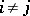(-additivity).

## Contents

### Examples of probability measures.

1)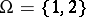;is the class of all subsets of;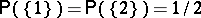(this probability measure corresponds to a random experiment consisting in throwing a symmetrical coin; if heads correspond to 1 while tails correspond to 2, the probability of throwing heads (tails) is 1/2);

2);is the class of all subsets of;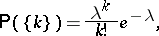where(the Poisson distribution);

3);is the class of Borel subsets of;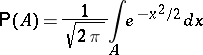(the normal distribution);

4)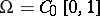is the space of continuous real functions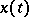on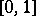that vanish at the point zero;is the class of Borel subsets with respect to the topology of uniform convergence;is the measure which is uniquely defined by the formula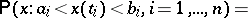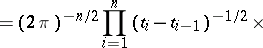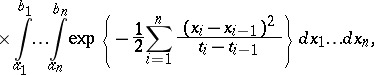whereis an arbitrary natural number and(the Wiener measure).

How to Cite This Entry:
Probability measure. Encyclopedia of Mathematics. URL: http://encyclopediaofmath.org/index.php?title=Probability_measure&oldid=11376
This article was adapted from an original article by V.V. Sazonov (originator), which appeared in Encyclopedia of Mathematics - ISBN 1402006098. See original article